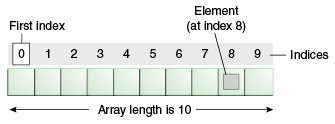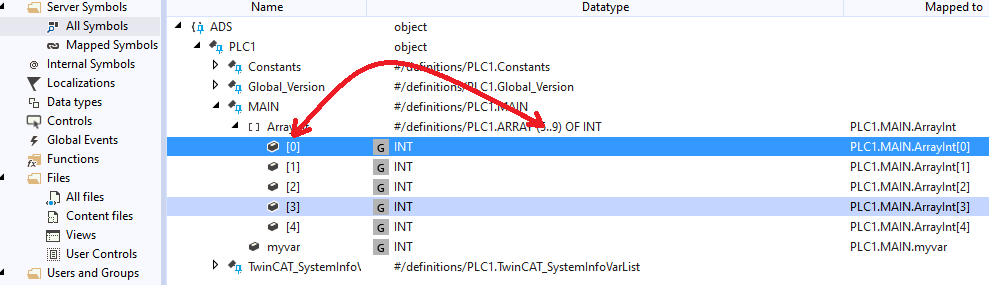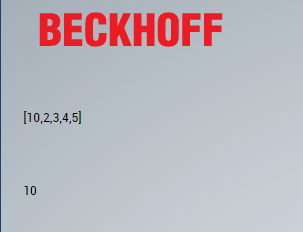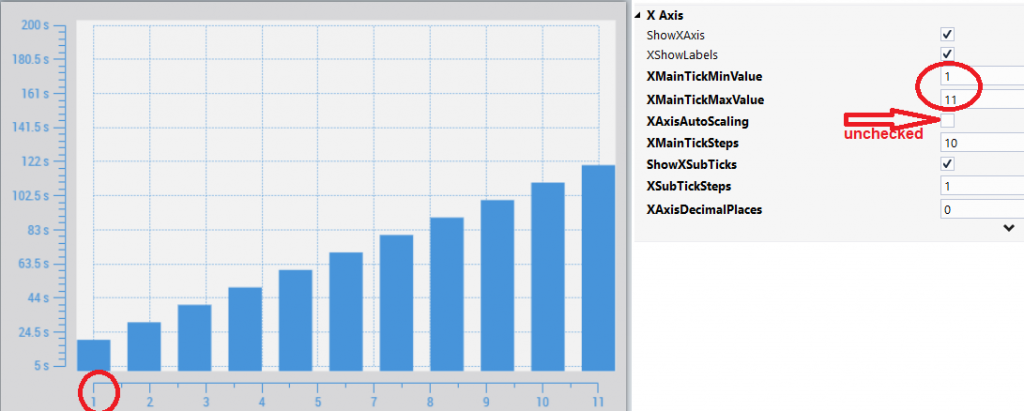## Array and Enumeration in Structured Text

What is an array:

An array can be defined as an ordered collection of items indexed by contiguous integers. An array is a data structure that can store a number of variables of the same data type in sequence. These similar elements could be of type int, float, double, char,  Function Block, etc.

Arrays are used when there is a need to use many variables of the same type. It can be defined as a sequence of objects which are of the same data type. It is used to store a collection of data, and it is more useful to think of an array as a collection of variables of the same type. Arrays can be declared and used. A programmer has to specify the types of elements and the number of elements that are required by an array. This is called a single-dimensional array. The array size should be an integer constant and greater than zero.

One-, two-, and three-dimensional fields (arrays) are supported as elementary data types. Arrays can be defined both in the declaration part of a POU and in the global variable lists.

In the following statement, we declare an array of 5 integers and initialized them with 1, 2, 3, 4, and 5 respectively.

`ArrayInt : ARRAY [1..5] OF INT := [1,2,3,4,5];`

Look at the syntax ARRAY[lower..upper] OF allows us to define any lower or upper bounds. As compared to C#, our lower bound doesn’t have to be zero. The total number of elements in the array is upper – lower + 1. We could have declared the above statement as the following as well

`ArrayInt : ARRAY [2..6] OF INT := [1,2,3,4,5];`

In this case, we have accessed first number by  by the following way.

`firstOne := ArrayInt; // 1`

Now we shall describe, how we can declare an array of FUNCTION_BLOCK

Sample function block

```FUNCTION_BLOCK MotorAC
VAR_INPUT
machineName : WSTRING :="Type A";
END_VAR

VAR_OUTPUT
machineAlarm : BOOL := FALSE;
END_VAR
VAR
maxRotationValue : REAL := 0.0;
rotationPerSeconds : INT := 0;
myTimer : TON ;
startMachine : BOOL := FALSE;
END_VAR```

We declare and initialize those array of 3 instances in the following way.

```ArrayMotorAc : ARRAY [1..3] OF MotorAC := [
(machineName:= "Motor_one"),
(machineName:= "Motor_two"),
(machineName:= "Motor_three")
];
```

Initialization can be done in the following way.

`FOR index := 1 TO number_of_motor DO    ArrayMotorAc[index].InitMachine();		END_FOR;`

## Arrays with variable length

We can pass pointer of data to a method and calculate the index as shown in the following example.

`FUNCTION FuncArrayTestByPointer : INTVAR_INPUT  pData           : POINTER TO INT;  nSize           : UDINT;END_VARVAR  pIndex      : POINTER TO INT;  nUpperIndex     : UDINT;  nIndex          : UDINT;END_VAR`
`FuncArrayTestByPointer := 0;nUpperIndex := nSize / SIZEOF(pIndex^);IF (nUpperIndex > 0) THEN  FOR nIndex := 0 TO (nUpperIndex - 1) DO    pIndex := pData + (nIndex * SIZEOF(pIndex^));    FuncArrayTestByPointer := FuncArrayTestByPointer + pIndex^;     END_FOREND_IF`

Example usage

`array    : ARRAY[2..6] OF INT := [16, 34, 4, 43, 35];Sum    : INT;Sum := FuncArrayTestByPointer(ADR(array), SIZEOF(array));`

Newer way use the variable array as defined in 3rd Edition of IEC 61131-3

In function blocks, functions or methods, arrays with variable length can be declared in the declaration section VAR_IN_OUT. The operators LOWER_BOUND and UPPER_BOUND can be used to determine the index limits of the array that is actually used at runtime. LOWER_BOUND returns the lower limit, UPPER_BOUND returns the upper limit.

`FUNCTION FuncArrayTestByPointerNewWay : INTVAR_IN_OUT  arrData    : ARRAY[*] OF INT;END_VARVAR  nIndex     : DINT;END_VARFuncArrayTestByPointerNewWay := 0;FOR nIndex := LOWER_BOUND(arrData, 1) TO UPPER_BOUND(arrData, 1) DO  FuncArrayTestByPointerNewWay := FuncArrayTestByPointerNewWay + arrData[nIndex];END_FORExample usagearray   : ARRAY[2..8] OF INT := [36, 34, 4, 43, 35, 2, 65];Sum    : INT;Sum := FuncArrayTestByPointerNewWay(array);`

## PLC Array index and HMI array index

We declare an array like the following.

`ArrayInt : ARRAY [5..9] OF INT := [10,2,3,4,5];myvar : INT := -1;END_VAR//bodymyvar := ArrayInt;`

We have mapped those variables and want to display the ArrayInt and myvar on the HMI, see the mapping in the following picture.Figure 01: ArrayInt is mapped as ArrayInt in HMI

As we see in the HMI it is mapped from 0 to 4 and in the PLC it is declared as 5 to 0. This is the default behavior. We can read the first item by the following code. Note that we are using ArrayInt not 5

`async function asyncFunctionCall() {try {    const myvar = await ReadPLCVariable('%s%PLC1.MAIN.ArrayInt%/s%');    var myTextBlockControl = TcHmi.Controls.get('TcHmiTextblock_1');    myTextBlockControl.setText(myvar);}catch(error) {    console.log(`ErrorCode = \${error.ErrorCode}`);    console.log(`ErrorText = \${error.ErrorText}`);  }}asyncFunctionCall();`Figure 02: Second text block shows the number as read by the above code

## Tips

=> Changing index in HMI

Index in PLC can have any index, we can adjust the index HMI in the following way.Some info related to variable length in array.

LOWER_BOUND(arrData, 1) returns as lower bound of the supplied array, and its type is DINT. We can know the number of items by substracting lower bound from the upper bound.